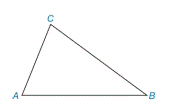Chapter 2.4, Problem 1E### Elementary Geometry for College St...

6th Edition
Daniel C. Alexander + 1 other
ISBN: 9781285195698

#### Solutions

Chapter
Section### Elementary Geometry for College St...

6th Edition
Daniel C. Alexander + 1 other
ISBN: 9781285195698
Textbook Problem
14 views

# In Exercise 1 to 4, refer to Δ A B C . On the basis of the information given, determine the measure of the remaining angle(s) of the triangle. m ∠ A = 63 ° and m ∠ B = 42 °To determine

To find:

The measure of C.

Explanation

Given:

The given statement is,

mA=63° and mB=42°

Figure (1)

Calculation:

In a triangle, the sum of the measures of the interior angles is 180°.

The given statement is,

mA=63° and mB=42°.

The sum of the measures of the interior angles is 180°

### Still sussing out bartleby?

Check out a sample textbook solution.

See a sample solution

#### The Solution to Your Study Problems

Bartleby provides explanations to thousands of textbook problems written by our experts, many with advanced degrees!

Get Started